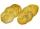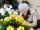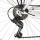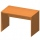# Prime numbers + greatest common divisor (GCD) - math problems

#### Number of problems found: 55

• DecomposeDecompose into primes and find the smallest common multiple n of (16,20) and the largest common divisor D of the pair of numbers (140,100)
• Common divisorsFind all common divisors of numbers 30 and 45.
• QuotientFind quotient before the bracket - the largest divisor 51 a + 34 b + 68 121y-99z-33
• LCD 2The least common denominator of 2/5, 1/2, and 3/4
• Diofant 2Is equation ? solvable on the set of integers Z?
• MO C–I–1 2018An unknown number is divisible by just four numbers from the set {6, 15, 20, 21, 70}. Determine which ones.
• Length of a stringWhat is the smallest length of a string that we can cut into 18 equal parts and even 27 equal parts (in decimeters)?
• SeedcakeSeedcake costs 44 cents. How many minimum seedcakes we must buy that we can pay in cash only whole euros?
• The ticketsThe tickets to the show cost some integer number greater than 1. Also, the sum of the price of the children's and adult tickets, as well as their product, was the power of the prime number. Find all possible ticket prices.
• FloristFlorist has 84 red and 48 white roses. How many same bouquets can he make from them if he must use all roses?
• Reminder and quotientThere are given the number C = 281, D = 201. Find the highest natural number S so that the C:S, D:S are with the remainder of 1,
• Bicycle wheelsDriving wheel of a bicycle has 54 teeth. The driven wheel has 22 teeth. After how many revolutions will meet the same teeth?
• TissuesThe store got three kinds of tissues - 132 children, 156 women and 204 men. Tissues each species were packed into boxes after the number of pieces the same for all three types (and greatest). Determine the number, if you know that every box has more than
• Largest squaresHow many of the largest square sheets did the plumber cut the honeycomb from 16 dm and 96 dm?
• School booksAt the beginning of the school year, the teacher distributed 480 workbooks and 220 textbooks. How many pupils could have the most in the classroom?
• ChairsIn the two dining rooms in the recreational building, there are equally arranged chairs around the tables. A maximum of 78 people can dine in the first dining room and 54 people in the second. How many chairs can be around one table?
• Diofant equationIn the set of integers (Z) solve the equation: ? Write result with integer parameter ? (parameter t = ...-2,-1,0,1,2,3... if equation has infinitely many solutions)
• Wide fieldThe field is 203 meters wide 319 meter long, what is the greater length of the rope by which length and width can be measured and find the exact number of time.
• The classroomThe classroom is 9 meters long. The width of the classroom is smaller and can be passed in equally long steps of 55 CM or 70 CM. Determine the width of the classroom.
• Ornamental shrubsChildren committed to plant 240 ornamental shrubs. Their commitment however exceeded by 48 shrubs. Write ratio of actually planted shrubs and commitment by lowest possible integers a/b.

Do you have an interesting mathematical word problem that you can't solve it? Submit a math problem, and we can try to solve it.

We will send a solution to your e-mail address. Solved examples are also published here. Please enter the e-mail correctly and check whether you don't have a full mailbox.

Please do not submit problems from current active competitions such as Mathematical Olympiad, correspondence seminars etc...

Do you want to calculate greatest common divisor two or more numbers? Prime numbers - math word problems. Greatest common divisor (GCD) - math word problems.